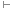# Hypothetical syllogism

A hypothetical syllogism is a valid argument form in logic.

The form of hypothetical syllogism is: “If P, then Q. If Q, then R. Therefore, if P, then R.” It may also be written as:

P → Q
Q → R
∴ P → R

P, Q and R may represent any proposition, or any other formula (using Greek letters to represent formulae rather than propositions, we may also express modus tollens as α → β, β → γα → γ).

## Examples of hypothetical syllogism

The following are examples of the hypothetical syllogism argument form:

If it rains, we will not have a picnic.
If we don't have a picnic, we won't need a picnic basket.
Therefore, if it rains, we won't need a picnic basket.

If the Montreal Canadiens win the Stanley Cup, I'll owe my dad some money.
If I owe my dad some money, I'll need to go to the bank.
So, if the Montreal Canadiens win the Stanley Cup, I'll need to go to the bank.